Скачать презентацию Lecture 3 How to value bonds and common

37798a5ff8272412fffa178b265208ed.ppt

• Количество слайдов: 32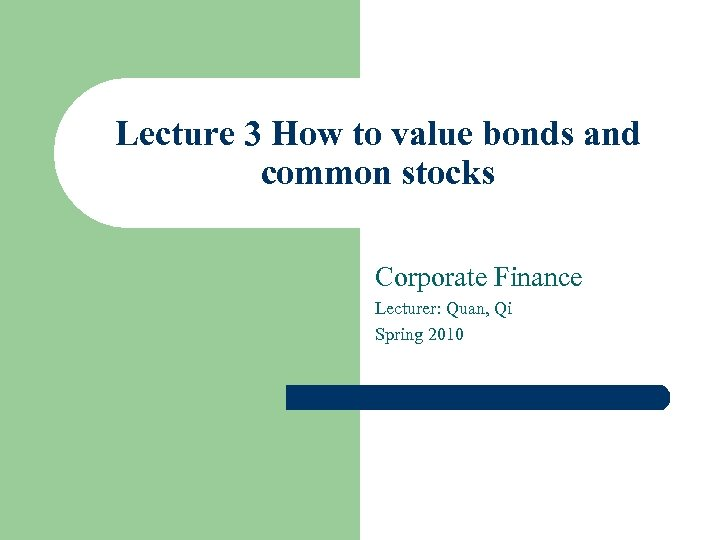Lecture 3 How to value bonds and common stocks Corporate Finance Lecturer: Quan, Qi Spring 2010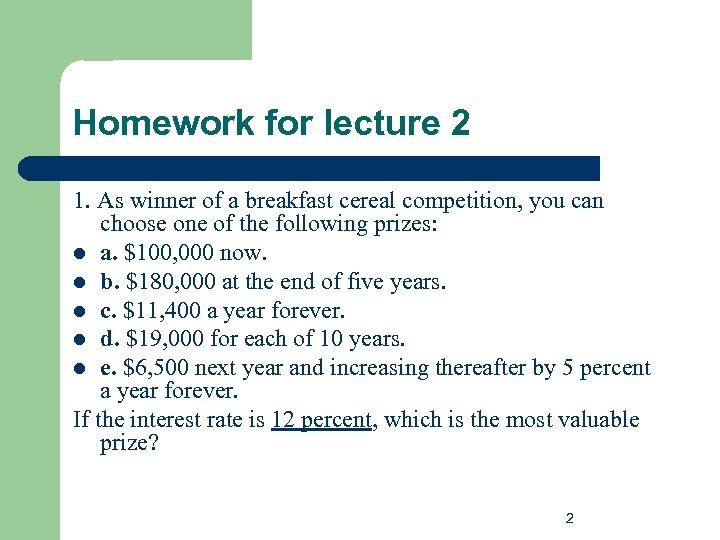Homework for lecture 2 1. As winner of a breakfast cereal competition, you can choose one of the following prizes: l a. \$100, 000 now. l b. \$180, 000 at the end of five years. l c. \$11, 400 a year forever. l d. \$19, 000 for each of 10 years. l e. \$6, 500 next year and increasing thereafter by 5 percent a year forever. If the interest rate is 12 percent, which is the most valuable prize? 2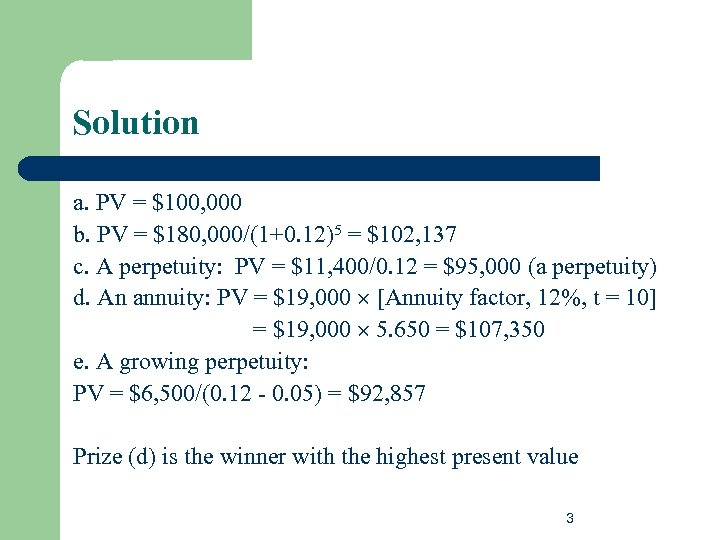Solution a. PV = \$100, 000 b. PV = \$180, 000/(1+0. 12)5 = \$102, 137 c. A perpetuity: PV = \$11, 400/0. 12 = \$95, 000 (a perpetuity) d. An annuity: PV = \$19, 000 [Annuity factor, 12%, t = 10] = \$19, 000 5. 650 = \$107, 350 e. A growing perpetuity: PV = \$6, 500/(0. 12 - 0. 05) = \$92, 857 Prize (d) is the winner with the highest present value 3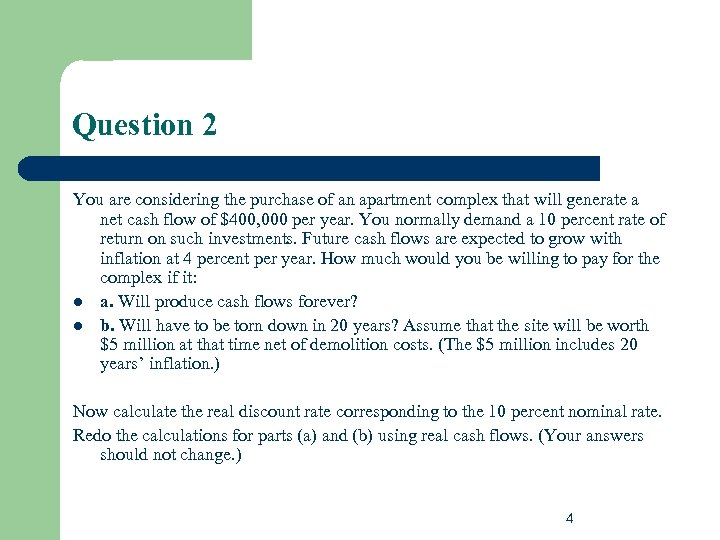Question 2 You are considering the purchase of an apartment complex that will generate a net cash flow of \$400, 000 per year. You normally demand a 10 percent rate of return on such investments. Future cash flows are expected to grow with inflation at 4 percent per year. How much would you be willing to pay for the complex if it: l a. Will produce cash flows forever? l b. Will have to be torn down in 20 years? Assume that the site will be worth \$5 million at that time net of demolition costs. (The \$5 million includes 20 years’ inflation. ) Now calculate the real discount rate corresponding to the 10 percent nominal rate. Redo the calculations for parts (a) and (b) using real cash flows. (Your answers should not change. ) 4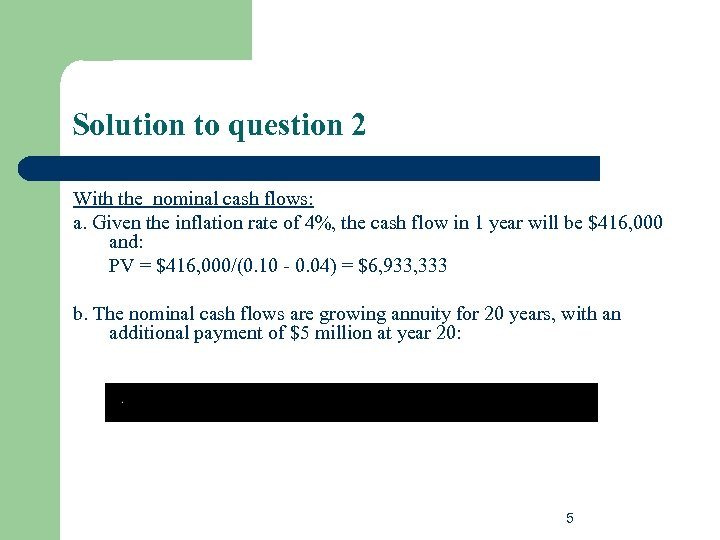Solution to question 2 With the nominal cash flows: a. Given the inflation rate of 4%, the cash flow in 1 year will be \$416, 000 and: PV = \$416, 000/(0. 10 - 0. 04) = \$6, 933, 333 b. The nominal cash flows are growing annuity for 20 years, with an additional payment of \$5 million at year 20: 5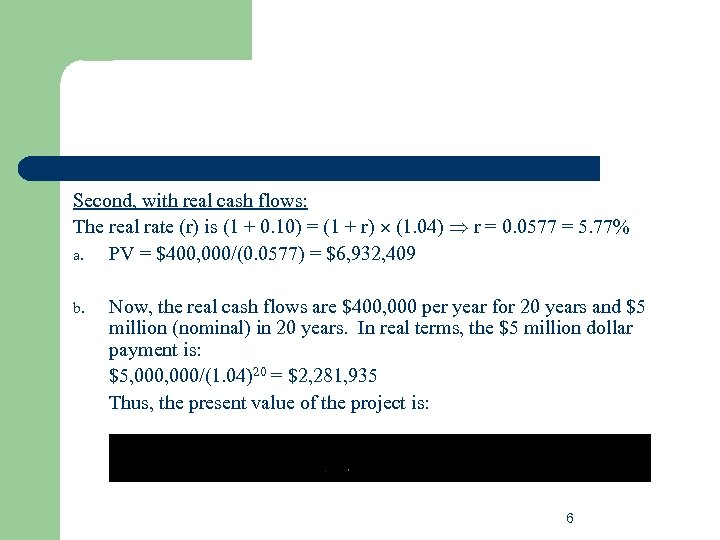Second, with real cash flows: The real rate (r) is (1 + 0. 10) = (1 + r) (1. 04) r = 0. 0577 = 5. 77% a. PV = \$400, 000/(0. 0577) = \$6, 932, 409 b. Now, the real cash flows are \$400, 000 per year for 20 years and \$5 million (nominal) in 20 years. In real terms, the \$5 million dollar payment is: \$5, 000/(1. 04)20 = \$2, 281, 935 Thus, the present value of the project is: 6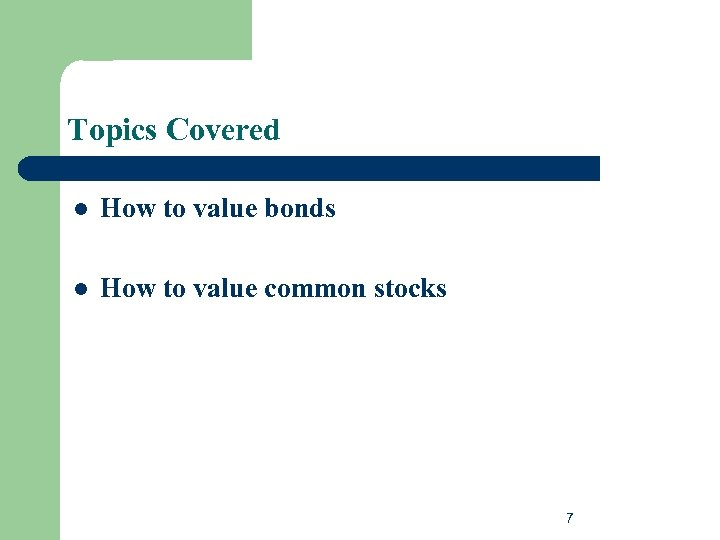Topics Covered l How to value bonds l How to value common stocks 7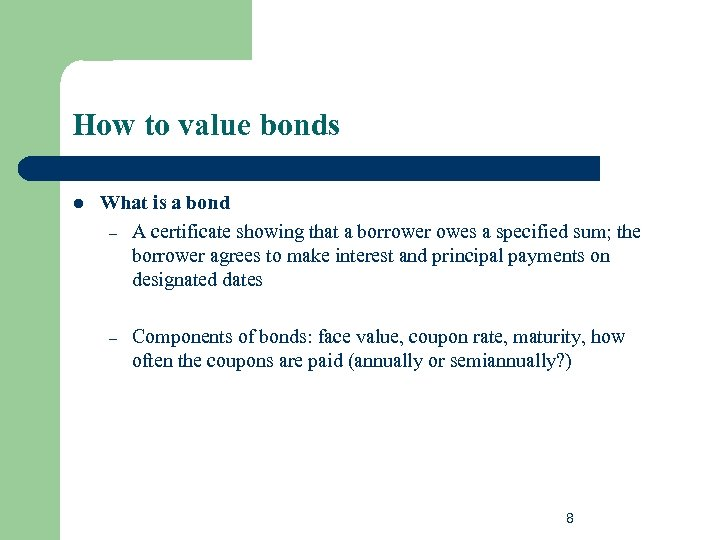How to value bonds l What is a bond – A certificate showing that a borrower owes a specified sum; the borrower agrees to make interest and principal payments on designated dates – Components of bonds: face value, coupon rate, maturity, how often the coupons are paid (annually or semiannually? ) 8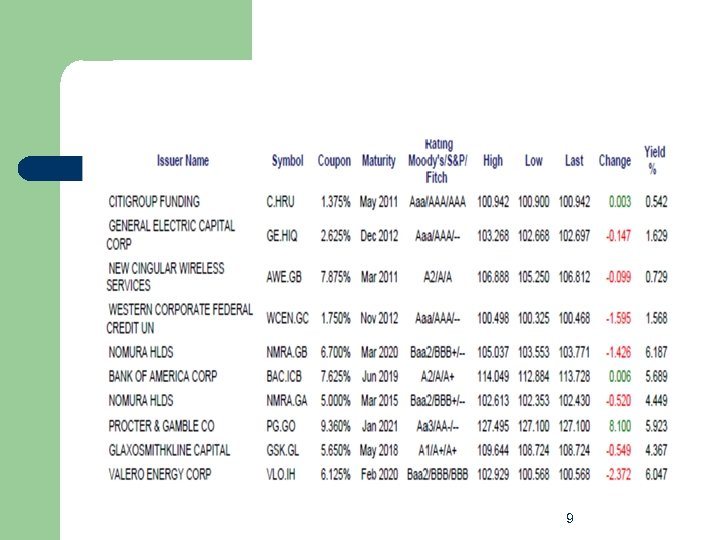9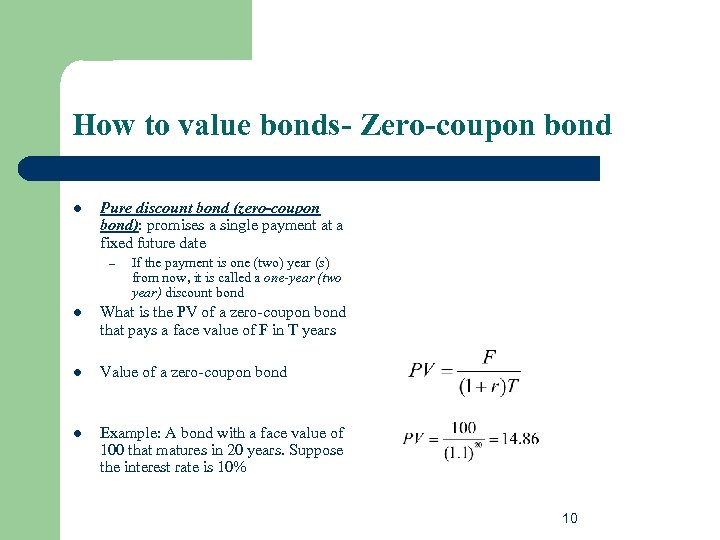How to value bonds- Zero-coupon bond l Pure discount bond (zero-coupon bond): promises a single payment at a fixed future date – If the payment is one (two) year (s) from now, it is called a one-year (two year) discount bond l What is the PV of a zero-coupon bond that pays a face value of F in T years l Value of a zero-coupon bond l Example: A bond with a face value of 100 that matures in 20 years. Suppose the interest rate is 10% 10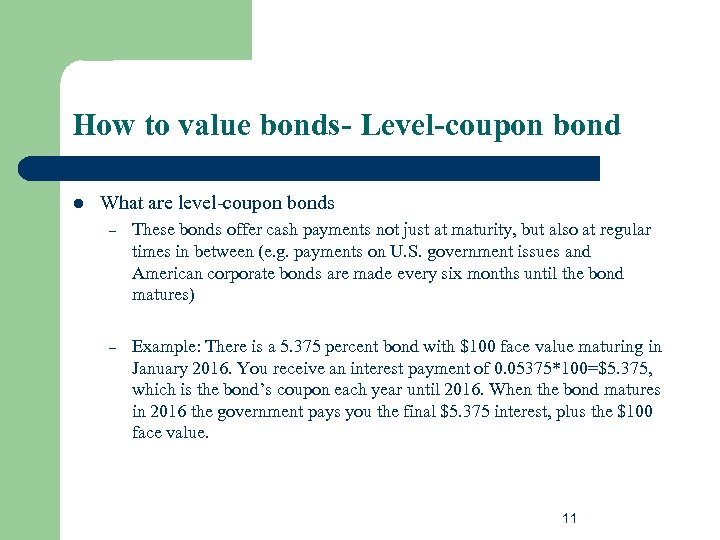How to value bonds- Level-coupon bond l What are level-coupon bonds – These bonds offer cash payments not just at maturity, but also at regular times in between (e. g. payments on U. S. government issues and American corporate bonds are made every six months until the bond matures) – Example: There is a 5. 375 percent bond with \$100 face value maturing in January 2016. You receive an interest payment of 0. 05375*100=\$5. 375, which is the bond’s coupon each year until 2016. When the bond matures in 2016 the government pays you the final \$5. 375 interest, plus the \$100 face value. 11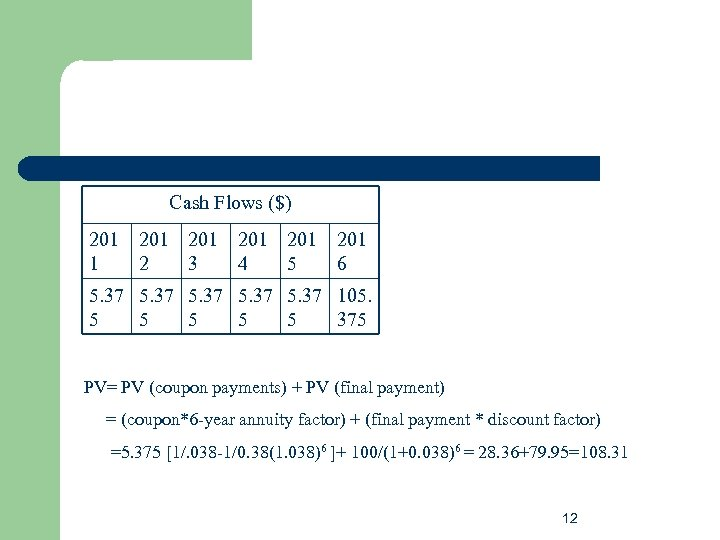Cash Flows (\$) 201 201 201 1 2 3 4 5 6 5. 37 105. 5 5 5 375 PV= PV (coupon payments) + PV (final payment) = (coupon*6 -year annuity factor) + (final payment * discount factor) =5. 375 [1/. 038 -1/0. 38(1. 038)6 ]+ 100/(1+0. 038)6 = 28. 36+79. 95=108. 31 12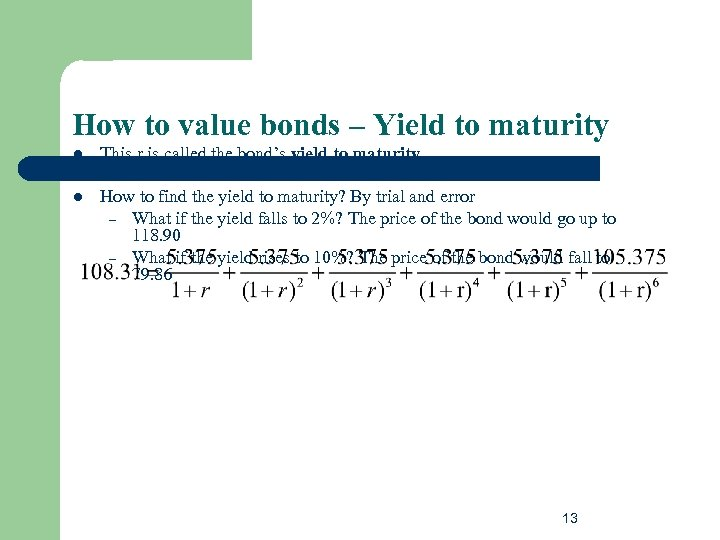How to value bonds – Yield to maturity l This r is called the bond’s yield to maturity l How to find the yield to maturity? By trial and error – What if the yield falls to 2%? The price of the bond would go up to 118. 90 – What if the yield rises to 10%? The price of the bond would fall to 79. 86 13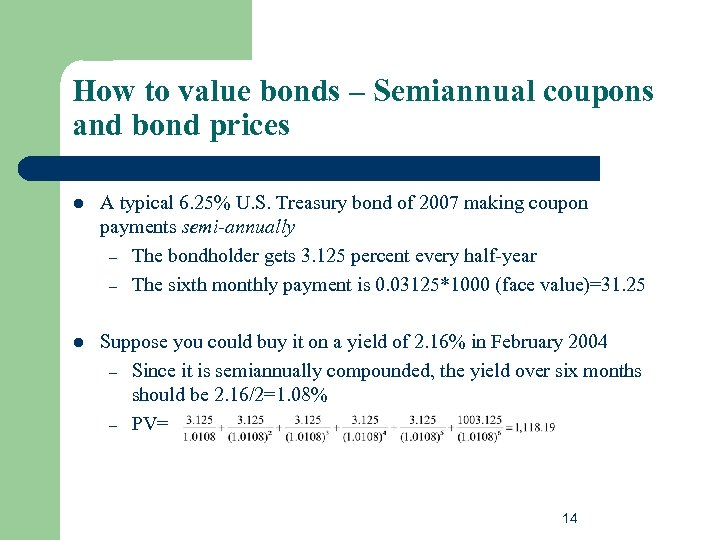How to value bonds – Semiannual coupons and bond prices l A typical 6. 25% U. S. Treasury bond of 2007 making coupon payments semi-annually – The bondholder gets 3. 125 percent every half-year – The sixth monthly payment is 0. 03125*1000 (face value)=31. 25 l Suppose you could buy it on a yield of 2. 16% in February 2004 – Since it is semiannually compounded, the yield over six months should be 2. 16/2=1. 08% – PV= 14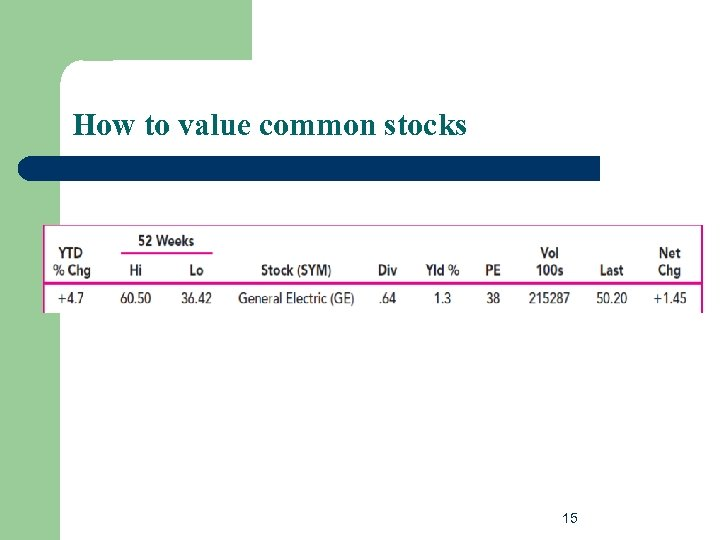How to value common stocks 15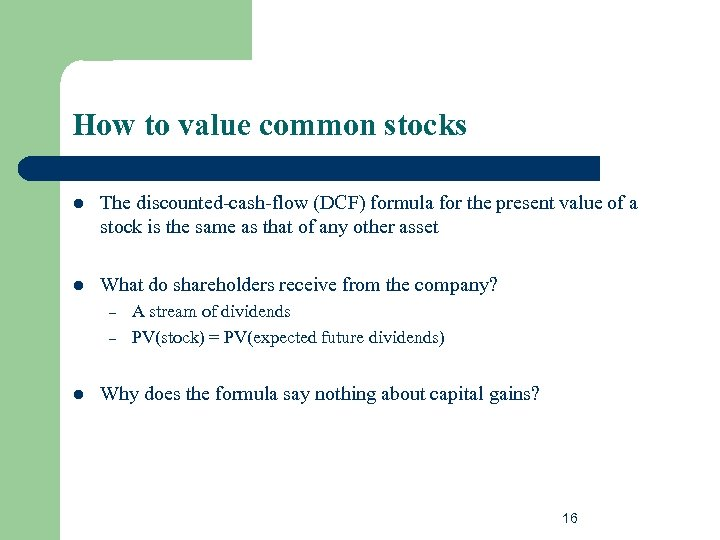How to value common stocks l The discounted-cash-flow (DCF) formula for the present value of a stock is the same as that of any other asset l What do shareholders receive from the company? – – l A stream of dividends PV(stock) = PV(expected future dividends) Why does the formula say nothing about capital gains? 16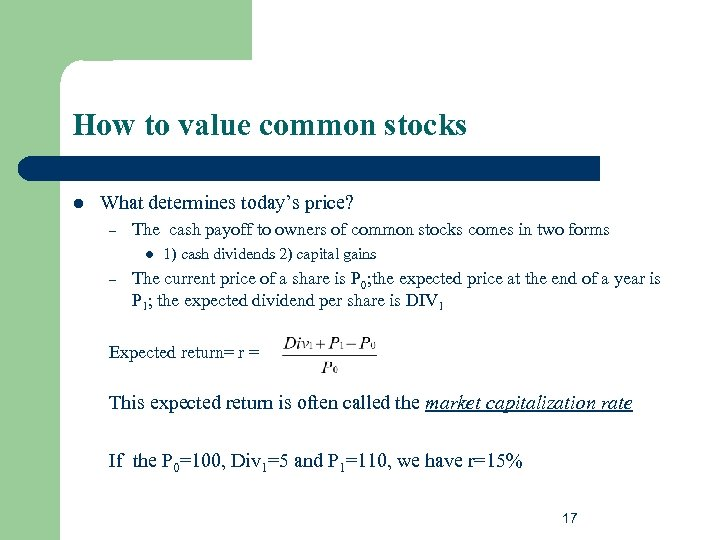How to value common stocks l What determines today’s price? – The cash payoff to owners of common stocks comes in two forms l – 1) cash dividends 2) capital gains The current price of a share is P 0; the expected price at the end of a year is P 1; the expected dividend per share is DIV 1 Expected return= r = This expected return is often called the market capitalization rate If the P 0=100, Div 1=5 and P 1=110, we have r=15% 17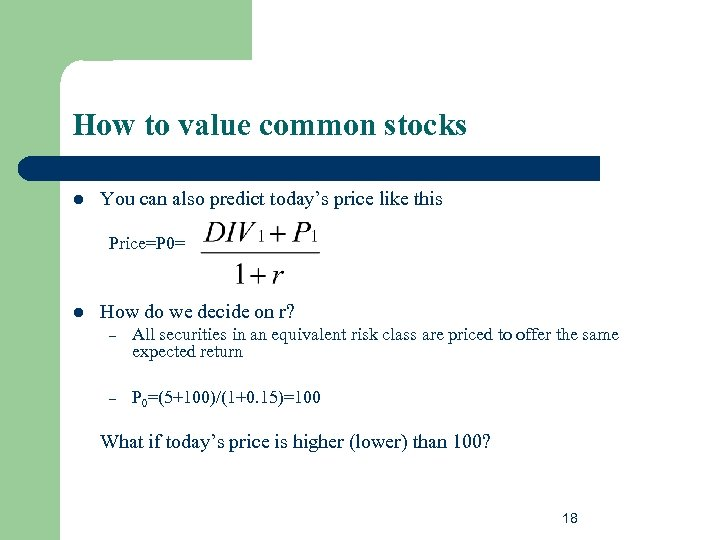How to value common stocks l You can also predict today’s price like this Price=P 0= l How do we decide on r? – All securities in an equivalent risk class are priced to offer the same expected return – P 0=(5+100)/(1+0. 15)=100 What if today’s price is higher (lower) than 100? 18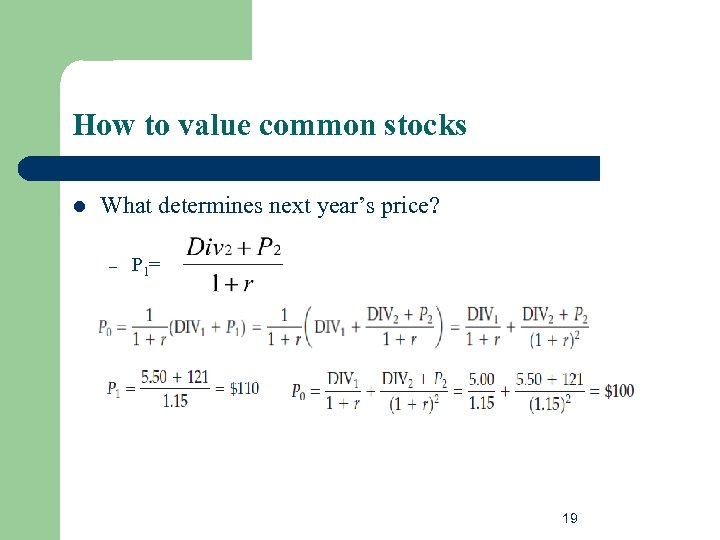How to value common stocks l What determines next year’s price? – P 1 = 19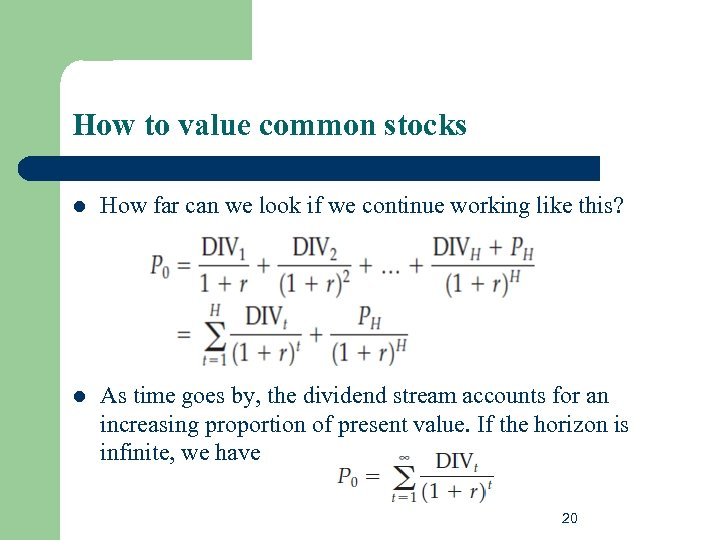How to value common stocks l How far can we look if we continue working like this? l As time goes by, the dividend stream accounts for an increasing proportion of present value. If the horizon is infinite, we have 20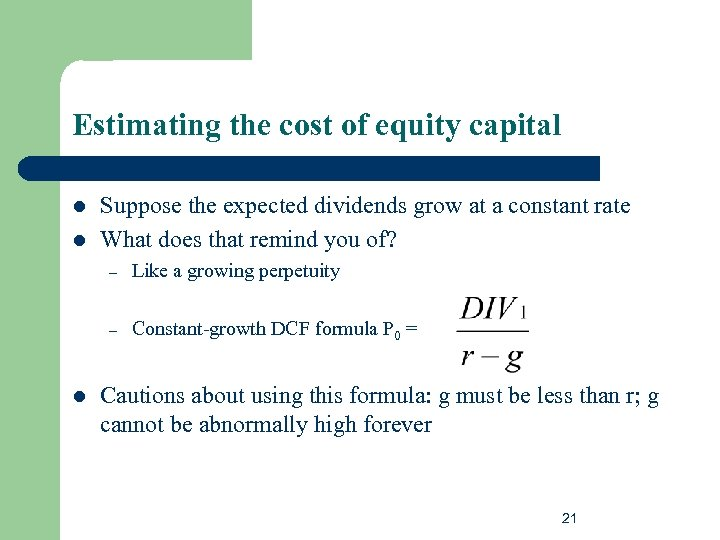Estimating the cost of equity capital l l Suppose the expected dividends grow at a constant rate What does that remind you of? – – l Like a growing perpetuity Constant-growth DCF formula P 0 = Cautions about using this formula: g must be less than r; g cannot be abnormally high forever 21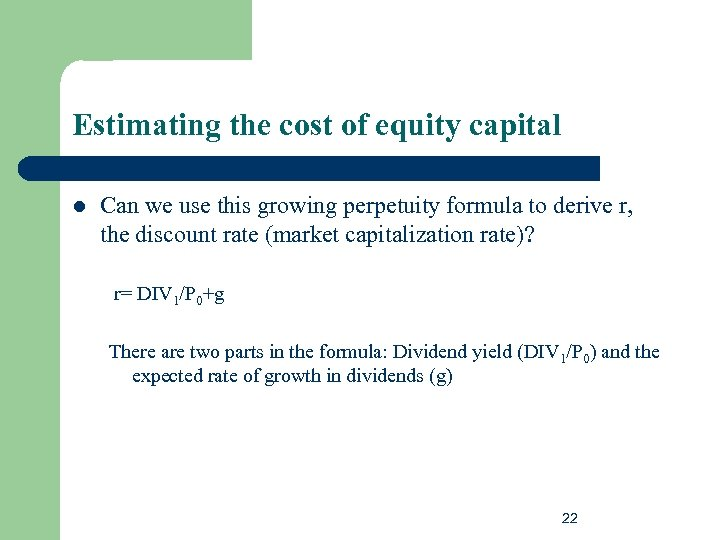Estimating the cost of equity capital l Can we use this growing perpetuity formula to derive r, the discount rate (market capitalization rate)? r= DIV 1/P 0+g There are two parts in the formula: Dividend yield (DIV 1/P 0) and the expected rate of growth in dividends (g) 22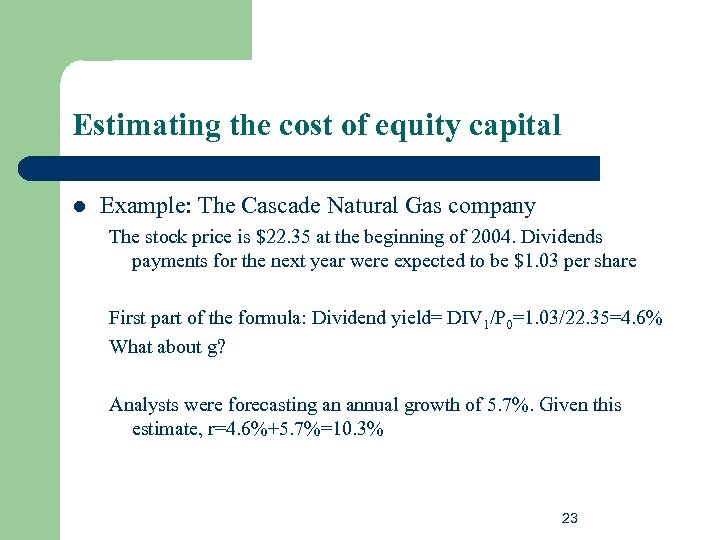Estimating the cost of equity capital l Example: The Cascade Natural Gas company The stock price is \$22. 35 at the beginning of 2004. Dividends payments for the next year were expected to be \$1. 03 per share First part of the formula: Dividend yield= DIV 1/P 0=1. 03/22. 35=4. 6% What about g? Analysts were forecasting an annual growth of 5. 7%. Given this estimate, r=4. 6%+5. 7%=10. 3% 23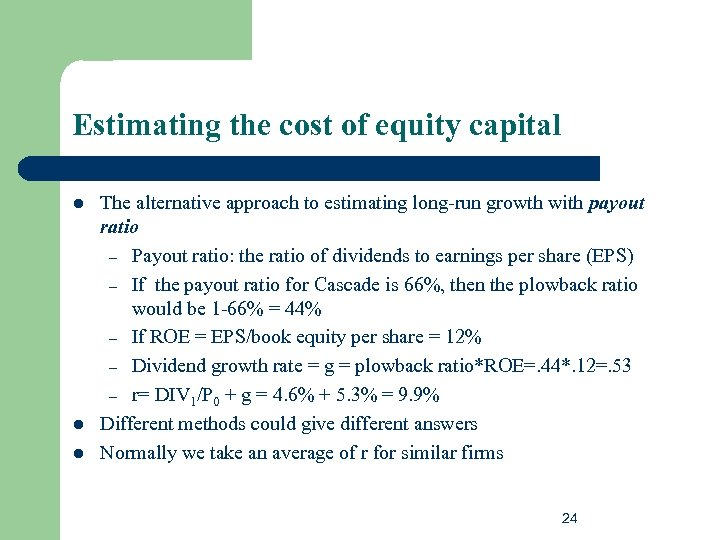Estimating the cost of equity capital l The alternative approach to estimating long-run growth with payout ratio – Payout ratio: the ratio of dividends to earnings per share (EPS) – If the payout ratio for Cascade is 66%, then the plowback ratio would be 1 -66% = 44% – If ROE = EPS/book equity per share = 12% – Dividend growth rate = g = plowback ratio*ROE=. 44*. 12=. 53 – r= DIV 1/P 0 + g = 4. 6% + 5. 3% = 9. 9% Different methods could give different answers Normally we take an average of r for similar firms 24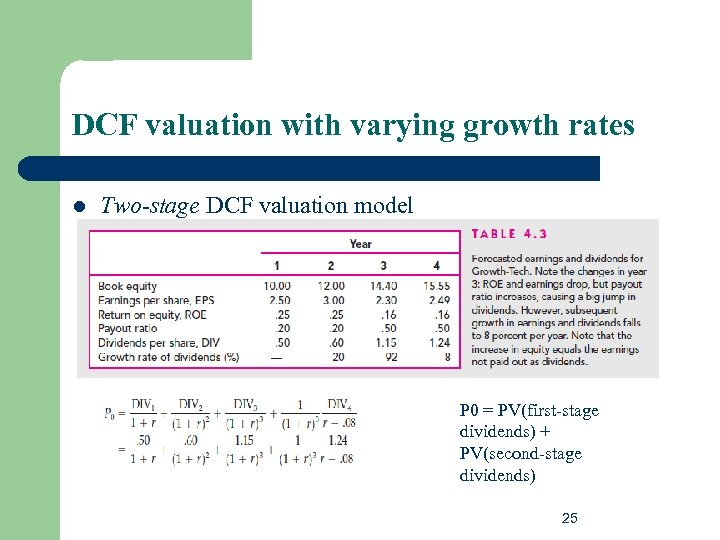DCF valuation with varying growth rates l Two-stage DCF valuation model P 0 = PV(first-stage dividends) + PV(second-stage dividends) 25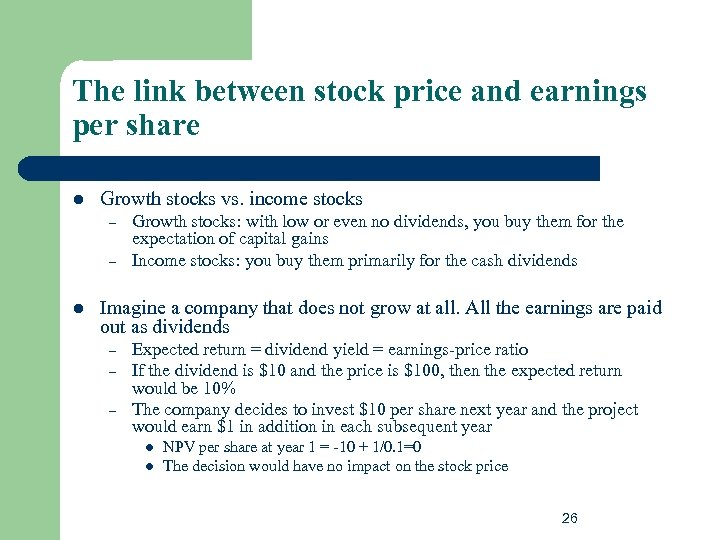The link between stock price and earnings per share l Growth stocks vs. income stocks – – l Growth stocks: with low or even no dividends, you buy them for the expectation of capital gains Income stocks: you buy them primarily for the cash dividends Imagine a company that does not grow at all. All the earnings are paid out as dividends – – – Expected return = dividend yield = earnings-price ratio If the dividend is \$10 and the price is \$100, then the expected return would be 10% The company decides to invest \$10 per share next year and the project would earn \$1 in addition in each subsequent year l l NPV per share at year 1 = -10 + 1/0. 1=0 The decision would have no impact on the stock price 26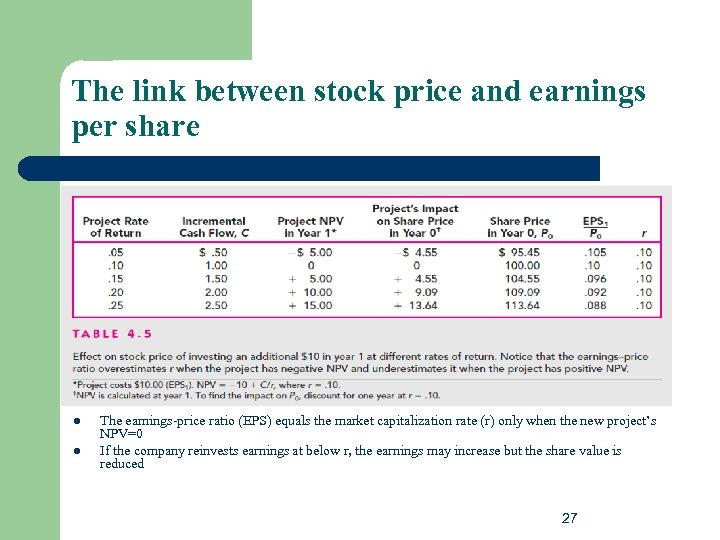The link between stock price and earnings per share l l The earnings-price ratio (EPS) equals the market capitalization rate (r) only when the new project’s NPV=0 If the company reinvests earnings at below r, the earnings may increase but the share value is reduced 27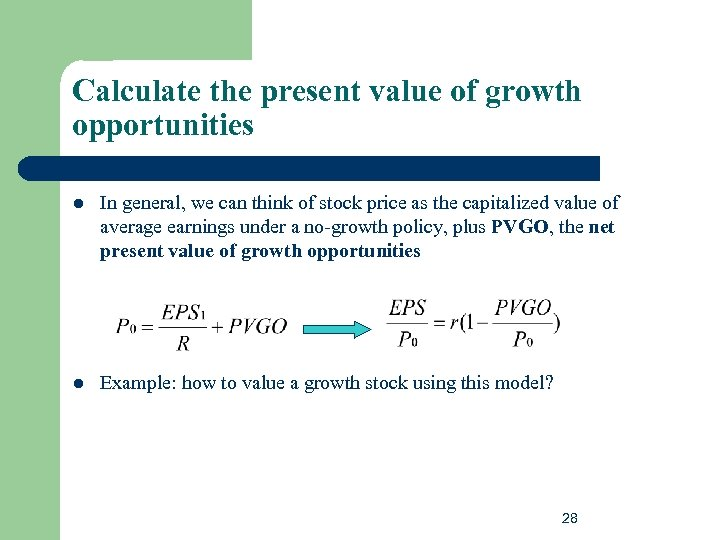Calculate the present value of growth opportunities l In general, we can think of stock price as the capitalized value of average earnings under a no-growth policy, plus PVGO, the net present value of growth opportunities l Example: how to value a growth stock using this model? 28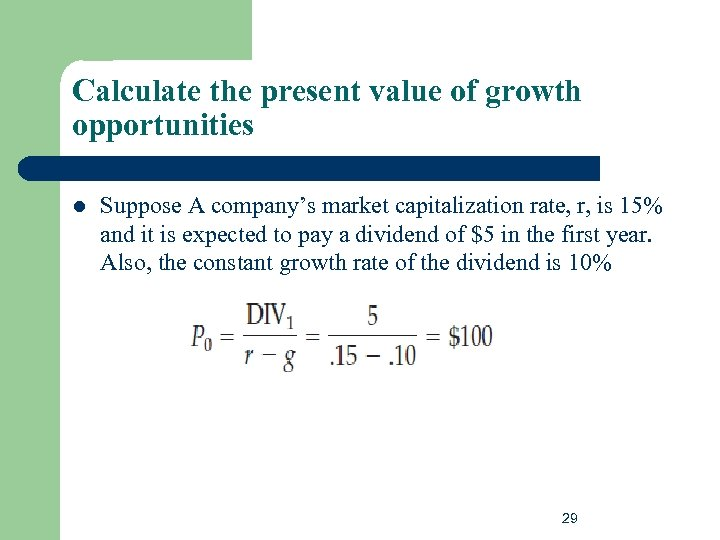Calculate the present value of growth opportunities l Suppose A company’s market capitalization rate, r, is 15% and it is expected to pay a dividend of \$5 in the first year. Also, the constant growth rate of the dividend is 10% 29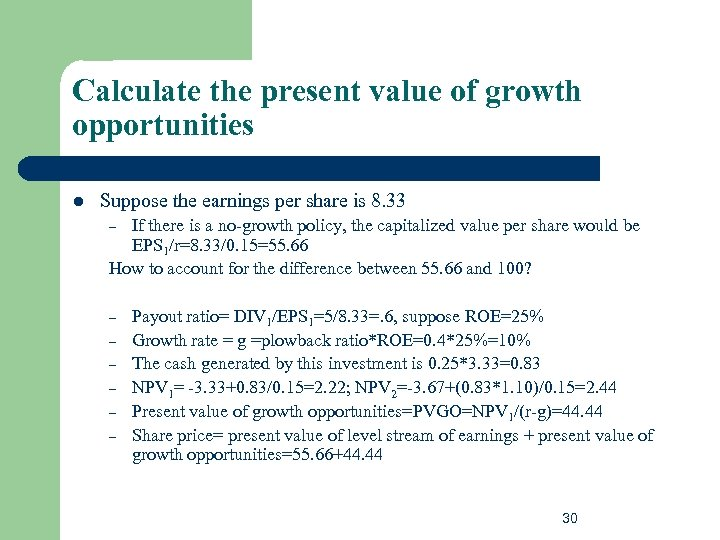Calculate the present value of growth opportunities l Suppose the earnings per share is 8. 33 If there is a no-growth policy, the capitalized value per share would be EPS 1/r=8. 33/0. 15=55. 66 How to account for the difference between 55. 66 and 100? – – – – Payout ratio= DIV 1/EPS 1=5/8. 33=. 6, suppose ROE=25% Growth rate = g =plowback ratio*ROE=0. 4*25%=10% The cash generated by this investment is 0. 25*3. 33=0. 83 NPV 1= -3. 33+0. 83/0. 15=2. 22; NPV 2=-3. 67+(0. 83*1. 10)/0. 15=2. 44 Present value of growth opportunities=PVGO=NPV 1/(r-g)=44. 44 Share price= present value of level stream of earnings + present value of growth opportunities=55. 66+44. 44 30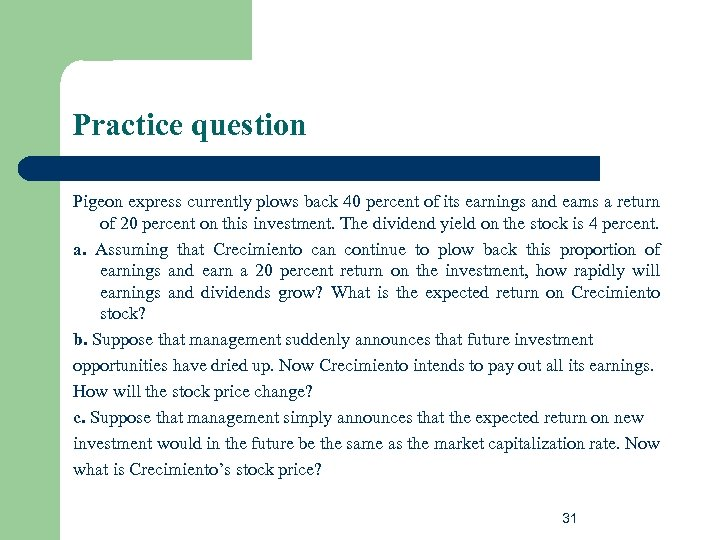Practice question Pigeon express currently plows back 40 percent of its earnings and earns a return of 20 percent on this investment. The dividend yield on the stock is 4 percent. a. Assuming that Crecimiento can continue to plow back this proportion of earnings and earn a 20 percent return on the investment, how rapidly will earnings and dividends grow? What is the expected return on Crecimiento stock? b. Suppose that management suddenly announces that future investment opportunities have dried up. Now Crecimiento intends to pay out all its earnings. How will the stock price change? c. Suppose that management simply announces that the expected return on new investment would in the future be the same as the market capitalization rate. Now what is Crecimiento’s stock price? 31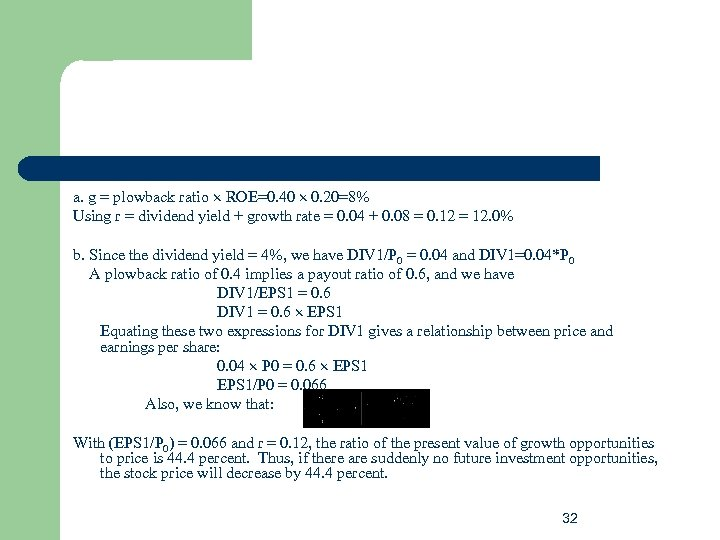a. g = plowback ratio ROE=0. 40 0. 20=8% Using r = dividend yield + growth rate = 0. 04 + 0. 08 = 0. 12 = 12. 0% b. Since the dividend yield = 4%, we have DIV 1/P 0 = 0. 04 and DIV 1=0. 04*P 0 A plowback ratio of 0. 4 implies a payout ratio of 0. 6, and we have DIV 1/EPS 1 = 0. 6 DIV 1 = 0. 6 EPS 1 Equating these two expressions for DIV 1 gives a relationship between price and earnings per share: 0. 04 P 0 = 0. 6 EPS 1/P 0 = 0. 066 Also, we know that: With (EPS 1/P 0) = 0. 066 and r = 0. 12, the ratio of the present value of growth opportunities to price is 44. 4 percent. Thus, if there are suddenly no future investment opportunities, the stock price will decrease by 44. 4 percent. 32